Amount of cuttings produced per foot of hole and total solid generated

After learning about capacity calculation, we can apply the capacity calculation to determine how much barrels of cutting produced per foot of hole drilled and total solid generated in pounds.

Use formula#1 and #2 for calculating amount of cutting generated per feet drilled.

Formula#1 for BARRELS of cuttings drilled per foot of hole drilled:

Barrels of cutting per foot drilled = Dh2 x (1 – % porosity) ÷1029.4

Where: Dh is hole diameter in inch.

Example: Determine barrels of cuttings drilled for one foot of 6-1/8 inch hole with 25% (0.25) porosity:

Barrels/footage drilled = 6.1252 x (1 – 0.25) ÷1029.4
Barrels/footage drilled = 0.02733 bbl/footage drilled

Formula#2 for CUBIC FEET of cuttings drilled per foot of hole drilled:

Cubic feet of cutting per foot drilled = Dh2 x 0.7854 x (1 – % porosity) ÷144

Where: Dh is hole diameter in inch.

Example: Determine barrels of cuttings drilled for one foot of 6-1/8 inch hole with 25% (0.25) porosity

Cubic feet/footage drilled = 6.1252 x 0.7854 x (1 – 0.25) ÷144
Cubic feet/footage drilled = 0.153462 cu ft/footage drilled

Moreover, you also apply sample density and volume relationship to determine total solids generated. Use the following formula to calculate total solid generated.

Wcg = 350 x Ch x L x (l – porosity) x Cutting density

Where;

Wcg = solids generated in pounds
Ch = capacity of hole in bbl/ft
L = footage drilled in ft
Cutting density = cutting density in gm/cc

Example: Determine the total pounds of solids generated in drilling 100 ft of  6-1/8 inch hole (0.03644 bbl/ft).

Density of cuttings = 2.20 gm/cc.

Porosity = 25%:
Wcg = 350 x 0.03644 x 100 x (1 – 0.25) x 2.2
Wcg = 2104.41 pounds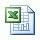Please find the excel sheet how to calculate how much cuttings drilled per foot of hole drilled and total solids generated

Ref books: Lapeyrouse, N.J., 2002. Formulas and calculations for drilling, production and workover, Boston: Gulf Professional publishing.

Bourgoyne, A.J.T., Chenevert , M.E. & Millheim, K.K., 1986. SPE Textbook Series, Volume 2: Applied Drilling Engineering, Society of Petroleum Engineers.

Mitchell, R.F., Miska, S. & Aadny, B.S., 2011. Fundamentals of drilling engineering, Richardson, TX: Society of Petroleum Engineers.

Pipe Displacement Calculation

Pipe displacement, normally in bbl/ft or m3/m, is steel volume  per length of steel pipe.  When we either pull out of hole or trip in hole for any kind of pipes such as drill pipe, casing or tubing, you should know how much fluid to displace steel volume.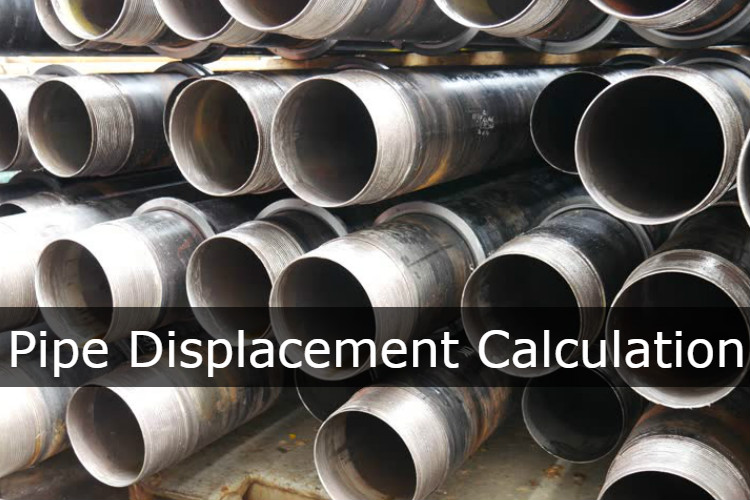For example, when we pull out of hole, a trip sheet must be monitored all time. We must know how much fluid will fill the hole each stand of drill pipe pulled out. If the volume of displacement less than theoretical displacement value, we may have problem due to swabbing formation into wellbore.

This article will demonstrate how to calculate plain pipe displacement with this following formula:

Oilfield Unit

Pipe Displacement in bbl/ft = (OD2 – ID2 ) ÷ 1029.4

Where;
OD in inch
ID in inch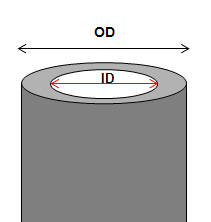Where,
OD is out side diameter of pipe in inch.
ID is inside diameter of pipe in inch.

This formula is for plain pipe diplacment such as casing and tubing. It is not for closed-end displacement. It’s not accurate enough for drill pipe because this formula does not account for tool joint displacement therefore you need drill pipe specification sheet for its displacement.

Example: Determine pipe displacement in bbl/ft of 9-5/8” casing 40 ppf, OD = 9.625 in, ID = 8.835 in

Pipe Displacement of 9-5/8” casing 40 ppf in bbl/ft = (9.6252 – 8.8352 ) ÷1029.4
Pipe Displacement of 9-5/8” casing 40 ppf in bbl/ft = 0.01417 bbl/ft

Metric Unit

Annular capacity in m3/m =  (OD2 – ID2) ÷1,273,240

Where;
OD in mm
ID in mm

Example: Determine pipe displacement in bbl/ft of 9-5/8” casing 40 ppf, OD = 244.475 mm, ID = 244.409 mm

Pipe Displacement of 9-5/8” casing 40 ppf in m3/m = (244.4752 – 244.4092 ) ÷1,273,240
Pipe Displacement of 9-5/8” casing 40 ppf in m3//m = 0.0074

Ref books:

Lapeyrouse, N.J., 2002. Formulas and calculations for drilling, production and workover, Boston: Gulf Professional publishing.

Bourgoyne, A.J.T., Chenevert , M.E. & Millheim, K.K., 1986. SPE Textbook Series, Volume 2: Applied Drilling Engineering, Society of Petroleum Engineers.

Mitchell, R.F., Miska, S. & Aadny, B.S., 2011. Fundamentals of drilling engineering, Richardson, TX: Society of Petroleum Engineers.

Calculate inner capacity of open hole/inside cylindrical objects (Internal Capacity Factor)

From the previous post, you learn how to calculate annular capacity and this article shows you how to use the same principle to calculate internal capacity factor of  open hole & inside cylindrical objects such as tubular, drill pipe, drill collars, tubing, casing, etc.There are several formulas to calculate inner capacity depending on unit of inner capacity required. The formula are listed below;

Calculate Internal Capacity Factor in bbl/ft

Inner Capacity in bbl/ft = (ID)2 ÷1029.4

Where;
Internal Diameter (ID) in inch

Example:

Determine Internal Capacity Factor in bbl/ft of a 6-1/8 in. hole:
Internal Capacity Factor in bbl/ft = 6.1252÷1029.4
Internal Capacity Factor in bbl/ft = 0. 0364 bbl/ft

Calculate Internal Capacity Factor in gal/ft

Inner Capacity in gal/ft = (ID in.)2 ÷24.51
Where;
Internal Diameter (ID) in inch

Example:

Determine Inner Capacity Factor in gal/ft of 6-1/8 in. hole:
Internal Capacity Factor in gal/ft = 6.1252÷ 24.51
Internal Capacity Factor in gal/ft = 1.53 gal/ft

Calculate Internal Capacity Factorin cu-meter/meter (m3/m)

Annular capacity in m3/m =  (ID2) ÷1,273,240

Where;
Internal Diameter (ID) in mm

Example:

Hole size (ID) = 155.56  mm.
Internal Capacity Factor in m3/m = (155.562 ) ÷1,273,240
Internal Capacity Factor in m3/m = 0.0190 m3/m

Calculate Internal Capacity Factorin liter/meter (l/m)

Annular capacity in m3/m =  (ID2) ÷1,273.24

Where;
Internal Diameter (ID) in mm

Example:

Hole size (ID) = 155.56  mm.
Internal Capacity Factor in l/m = (155.562 ) ÷1,273.24
Internal Capacity Factor in l/m = 19.0 l/m

Volume can be determined by this following formula;

Oilfield Unit

Volume = Internal Capacity Factor x Length

Where;

Volume in bbl

Internal Capacity Factor in bbl/ft

Length in ft

Example:

Internal Capacity Factor =  0. 0364 bbl/ft

Hole Length = 3,000 ft

Volume = 0. 0364 x 3,000 = 109.2 bbl.

Metric Unit

Volume = Internal Capacity Factor x Length

Where;

Volume in cu.meter

Internal Capacity Factor in cu.meter/meter

Length in meter

Example:

Internal Capacity Factor in m3/m = 0.0190 m3/m

Hole Length = 3,000 m

Volume = 0. 0190 x 3,000 = 57 m3Please find the excel sheet on how to calculate internal capacity factor.

Ref books:

Lapeyrouse, N.J., 2002. Formulas and calculations for drilling, production and workover, Boston: Gulf Professional publishing.

Bourgoyne, A.J.T., Chenevert , M.E. & Millheim, K.K., 1986. SPE Textbook Series, Volume 2: Applied Drilling Engineering, Society of Petroleum Engineers.

Mitchell, R.F., Miska, S. & Aadny, B.S., 2011. Fundamentals of drilling engineering, Richardson, TX: Society of Petroleum Engineers.

Calculate Annular Capacity

Annular capacity  is volume of fluid between two diameter of cylindrical objects per length or length per volume. This article demonstrates you how to calculate annular capacity between casing or hole and drill pipe, tubing, or casing. There are several formulas as shown below to calculate annular capacity depending on unit of annular capacity required.Note: Dh is bigger ID and Dp is smaller OD. The examples below will show the Dh as hole size and Dp is drill pipe OD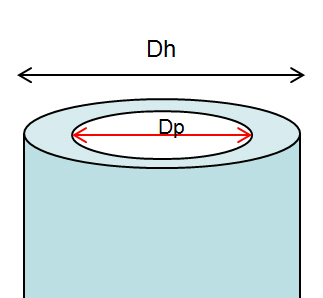a) Calculate annular capacity in bbl/ft

Annular capacity in bbl/ft =  (Dh2 – Dp2) ÷1029.4

Where;
Dh in inch
Dp in inch

Example: Hole size (Dh) = 6-1/8 in.
Drill pipe OD (Dp) = 3.5  in.
Annular capacity in bbl/ft = (6.1252 – 3.5 2) ÷1029.4
Annular capacity = 0.0245 bbl/ft

b) Calculate annular capacity in gal/ft

Annular capacity in gal/ft = (Dh2 – Dp2) ÷ 24.51

Where;
Dh in inch
Dp in inch

Example:
Hole size (Dh) = 6-1/8 in.
Drill pipe OD (Dp) = 3.5  in.
Annular capacity in gal/ft = (6.125 2 – 3.52) ÷24.51
Annular capacity = 1.031 gal/ft

c) Calculate annular capacity in cu-meter/meter (m3/m)

Annular capacity in m3/m =  (Dh2 – Dp2) ÷1,273,240

Where;
Dh in mm
Dp in mm

Example:
Hole size (Dh) = 155.56 mm.
Drill pipe OD (Dp) = 88.90  mm.
Annular capacity in m3/m = (155.562 – 88.90 2) ÷1,273,240
Annular capacity = 0.0128 m3/m

d) Calculate annular capacity in litre/meter (l/m)

Annular capacity in m3/m =  (Dh2 – Dp2) ÷1,273.24

Where;
Dh in mm
Dp in mm

Example:
Hole size (Dh) = 155.56 mm.
Drill pipe OD (Dp) = 88.90  mm.
Annular capacity in m3/mt = (155.562 – 88.90 2) ÷1,273.24
Annular capacity =12.8 l/m

Annular volume can be determined by this following formula;

Oilfield Unit

Annular volume in bbl  = annular capacity (bbl/ft) x length of annulus (ft)

Example:

Annular capacity = 0.0245 bbl/ft
Length of annulus = 1000 ft
Annular volume = 1000 x 0.0245 = 24.5 bbl.

Metric Unit

Annular volume in m3 = annular capacity (m3/m) x length of annulus (m)

Example:

Annular capacity = 0.0128 m3/m
Length of annulus = 330 m
Annular volume = 330 x 0.0128 = 4.224 m3.

** Please remember that if you have several annular profile, you must calculate volume based on each annular profile in order to get total annular volume.This is 2020 update with Metric Unit – Please find the Excel sheet for calculating annular capacity.

Ref books:

Lapeyrouse, N.J., 2002. Formulas and calculations for drilling, production and workover, Boston: Gulf Professional publishing.

Bourgoyne, A.J.T., Chenevert , M.E. & Millheim, K.K., 1986. SPE Textbook Series, Volume 2: Applied Drilling Engineering, Society of Petroleum Engineers.

Mitchell, R.F., Miska, S. & Aadny, B.S., 2011. Fundamentals of drilling engineering, Richardson, TX: Society of Petroleum Engineers.

Calculate Equivalent Circulation Density (ECD) with complex engineering equations

These formulas below are used for complex calculation for annular pressure loss and equivalent circulating density. I think this calculation will give you more accurate result than a simple equation. Please follow the following steps how to calculate annular pressure loss and ECD.

1. Determine n: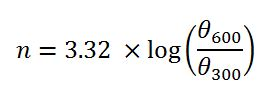2. Determine K: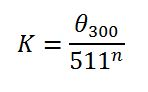3. Determine annular velocity (v) in ft/min: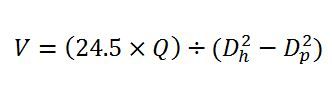4. Determine critical velocity (Vc) in ft/min: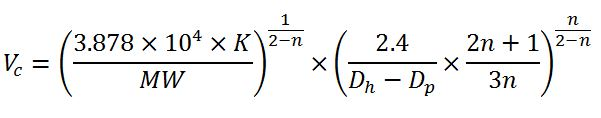5. Pressure loss for laminar flow (Ps), psi:6. Pressure loss for turbulent flow (Ps), psi:7. Determine equivalent circulating density (ECD), ppg: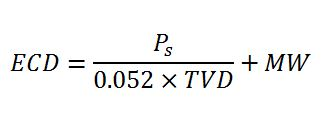Abbreviation meaning

θ300: viscometer dial reading at 300 rpm
θ600: viscometer dial reading at 600 rpm
Q: Flow rate in gpm
Dh: Diameter of hole
Dp: Diameter of drill pipe, drill collar or BHA in ft
v: annular velocity in ft/min
L: length of drill pipe, drill collar or BHA in ft
MW: Mud Weight
PV: Plastic viscosity

Example: Equivalent circulating density (ECD) in ppg by using following data:

Mud weight = 9.5 ppg
θ300 = 40
θ600 = 60
Plastic viscosity = 20 cps
Circulation rate = 650 gpm
Hole diameter = 8.5 in.
Drill collar OD = 6.75 in.
Drill pipe OD = 5.0 in
Drill collar length = 600 ft
Drill pipe length = 10,000 ft
True vertical depth = 9,000 ft

1. Determine n: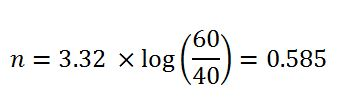2. Determine K: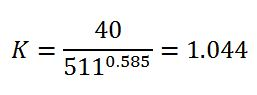3. Determine annular velocity (v) in ft/min around drill pipe: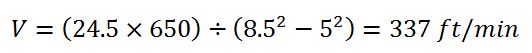4. Determine critical velocity (Vc) in ft/min around drill pipe: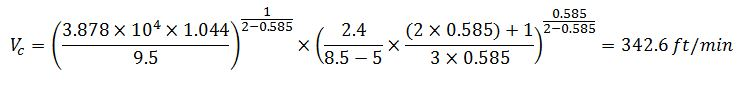The annular velocity around drill pipe is less than the critical velocity around drill pipe so this is laminar flow. The equation #5 (for laminar flow) must be applied in this case.

Pressure loss for turbulent flow (Ps), psi:5. Determine annular velocity (v) in ft/min around drill collar: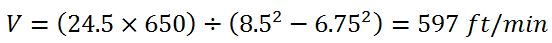6. Determine critical velocity (Vc) in ft/min around drill collar: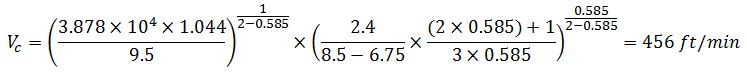The annular velocity around drill collar is more than the critical velocity around drill collar so this is turbulent flow. The equation #6 (for turbulent flow) must be applied in this case.

Pressure loss for laminar flow (Ps), psi:Total annular pressure loss = annular pressure loss around drill pipe + annular pressure loss around drill collar

Ps=271.3+81.5 = 352.8psi

7. Determine equivalent circulating density (ECD), ppg:Please find the Excel sheet for calculating ECD (engineering calculation)

Ref books: Lapeyrouse, N.J., 2002. Formulas and calculations for drilling, production and workover, Boston: Gulf Professional publishing.

Bourgoyne, A.J.T., Chenevert , M.E. & Millheim, K.K., 1986. SPE Textbook Series, Volume 2: Applied Drilling Engineering, Society of Petroleum Engineers.

Mitchell, R.F., Miska, S. & Aadny, B.S., 2011. Fundamentals of drilling engineering, Richardson, TX: Society of Petroleum Engineers.